Demo problem: A one-dimensional Poisson problem

In this document, we demonstrate how a solve the 1D Poisson problem using existing objects from the oomph-lib library:

 One-dimensional model Poisson problem Solve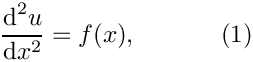in a one-dimensional domain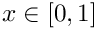, with boundary conditions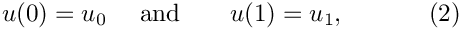where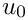and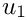are given.

We provide a detailed discussion of the driver code one_d_poisson.cc which solves the problem for the specific source function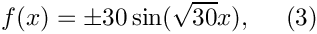and boundary conditions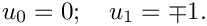In this case, the problem has the (fish-shaped) exact solution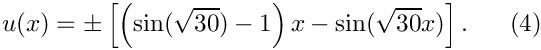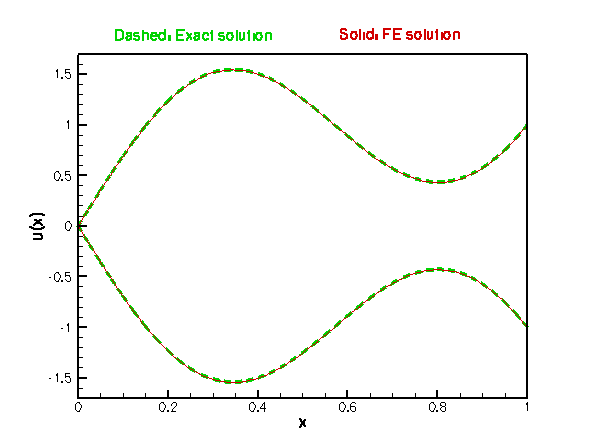The fish-shaped solution u(x).

# Global parameters and functions

Source functions and problem parameters generally need to be accessible to a variety of oomph-lib objects and we tend to define such functions/quantities in C++ namespaces. Here we use the namespace FishSolnOneDPoisson to define the source function (3) and the exact solution (4). Both functions use the integer value FishSolnOneDPoisson::Sign, which can be set by the "user" in the driver code.

//==start_of_namespace================================================
/// Namespace for fish-shaped solution of 1D Poisson equation
//====================================================================
{
/// Sign of the source function
/// (- gives the upper half of the fish, + the lower half)
int Sign=-1;
/// Exact, fish-shaped solution as a 1D vector
void get_exact_u(const Vector<double>& x, Vector<double>& u)
{
u = double(Sign)*((sin(sqrt(30.0))-1.0)*x-sin(sqrt(30.0)*x));
}
/// Source function required to make the fish shape an exact solution
void source_function(const Vector<double>& x, double& source)
{
source = double(Sign)*30.0*sin(sqrt(30.0)*x);
}
} // end of namespace
Namespace for fish-shaped solution of 1D Poisson equation.
int Sign
Sign of the source function (- gives the upper half of the fish, + the lower half)
void get_exact_u(const Vector< double > &x, Vector< double > &u)
Exact, fish-shaped solution as a 1D vector.
void source_function(const Vector< double > &x, double &source)
Source function required to make the fish shape an exact solution.

# The driver code

In order to solve the Poisson problem with oomph-lib, we represent the mathematical problem defined by equations (1) and (2) in a specific Problem object, OneDPoissonProblem. oomph-lib provides a variety of 1D Poisson elements (1D elements with linear, quadratic and cubic representations for the unknown function) and we pass the specific element type as a template parameter to the Problem. In the driver code, listed below, we use the QPoissonElement<1,4>, a four-node (cubic) 1D Poisson element. We pass the number of elements in the mesh as the first argument to the Problem constructor and the pointer to the source function, defined in the namespace FishSolnOneDPoisson, as the second.

Once the problem has been created, we execute Problem::self_test(), which checks that the Problem has been properly set up. If this test is passed, we proceed to solve the problem: Initially, we set the sign of the source function (defined in the variable FishSolnOneDPoisson::Sign) to -1 and solve, using oomph-lib's Newton solver. We write the solution to output files, using the OneDPoissonProblem's member function doc_solution(), discussed below. We then repeat the process, using a positive sign for the source function.

//======start_of_main==================================================
/// Driver for 1D Poisson problem
//=====================================================================
int main()
{
// Set up the problem:
// Solve a 1D Poisson problem using a source function that generates
// a fish shaped exact solution
unsigned n_element=40; //Number of elements
OneDPoissonProblem<QPoissonElement<1,4> > //Element type as template parameter
// Check whether the problem can be solved
cout << "\n\n\nProblem self-test ";
if (problem.self_test()==0)
{
cout << "passed: Problem can be solved." << std::endl;
}
else
{
throw OomphLibError("failed!",
OOMPH_CURRENT_FUNCTION,OOMPH_EXCEPTION_LOCATION);
}
// Set the sign of the source function:
cout << "\n\n\nSolving with negative sign:\n" << std::endl;
// Solve the problem with this Sign
problem.newton_solve();
//Output solution for this case (label output files with "0")
problem.doc_solution(0);
// Change the sign of the source function:
cout << "\n\n\nSolving with positive sign:\n" << std::endl;
// Re-solve the problem with this Sign (boundary conditions get
// updated automatically when Problem::actions_before_newton_solve() is
// called.
problem.newton_solve();
//Output solution for this case (label output files with "1")
problem.doc_solution(1);
} // end of main
1D Poisson problem in unit interval.
int main()
///////////////////////////////////////////////////////////////////// ///////////////////////////////...

# The problem class

oomph-lib driver codes tend to be very compact and "high-level" because all the "hard work" is done in the Problem specification. For our simple Poisson problem, this step is completely straightforward:

The OneDPoissonProblem is derived from oomph-lib's generic Problem class and, as discussed above, the specific element type is specified as a template parameter to make it easy for the "user" to change the element type in the driver code.

//==start_of_problem_class============================================
/// 1D Poisson problem in unit interval.
//====================================================================
template<class ELEMENT>
class OneDPoissonProblem : public Problem

The OneDPoissonProblemclass has five member functions, only three of which are non-trivial:

• the constructor OneDPoissonProblem(...)
• the function actions_before_newton_solve()
• the function doc_solution(...)

The function Problem::actions_after_newton_solve() is a pure virtual member function of the Problem base class and must be provided. However, it is not required in the present problem so we leave it empty. Similarly, the problem destructor can remain empty as all memory de-allocation is handled in the destructor of the Problem base class. The Problem only stores one private data member, the pointer to the source function.

public:
/// Constructor: Pass number of elements and pointer to source function
OneDPoissonProblem(const unsigned& n_element,
PoissonEquations<1>::PoissonSourceFctPt source_fct_pt);
/// Destructor (empty)
{
delete mesh_pt();
}
/// Update the problem specs before solve: (Re)set boundary conditions
/// Update the problem specs after solve (empty)
/// Doc the solution, pass the number of the case considered,
/// so that output files can be distinguished.
void doc_solution(const unsigned& label);
private:
/// Pointer to source function
PoissonEquations<1>::PoissonSourceFctPt Source_fct_pt;
}; // end of problem class
PoissonEquations< 1 >::PoissonSourceFctPt Source_fct_pt
Pointer to source function.
void actions_before_newton_solve()
Update the problem specs before solve: (Re)set boundary conditions.
~OneDPoissonProblem()
Destructor (empty)
void doc_solution(const unsigned &label)
Doc the solution, pass the number of the case considered, so that output files can be distinguished.
void actions_after_newton_solve()
Update the problem specs after solve (empty)
OneDPoissonProblem(const unsigned &n_element, PoissonEquations< 1 >::PoissonSourceFctPt source_fct_pt)
Constructor: Pass number of elements and pointer to source function.
 A general convention The type PoissonEquations<1>::PoissonSourceFctPt, used to define the type of the source function pointer, is a public typedef, defined in oomph-lib's Poisson equation class, as follows: [...] public: /// \short Function pointer to source function has the form fct(x,f(x)). /// Note that x is a Vector! typedef void (*PoissonSourceFctPt)(const Vector& x, double& f); [...] This reflects a general convention in oomph-lib: The function types of source functions etc. that are required by specific elements, are always declared as public types in the element classes. The logic behind this is that only the element writer/maintainer knows what type of function (i.e. the type of its arguments and its return value) a specific element requires. For instance, the source function for the Poisson equation requires the spatial coordinate x as input and computes the (scalar) value of the source function. Since the syntax for C++ function pointers is somewhat "non-obvious" (that's according to Bjarne Stroustrup, the designer of C++, himself!), we use typedefs to give the function pointers more intuitive names. Since the typedefs are public, they can be used anywhere in the "user's" code. While we're at it, here's another convention: If an oomph-lib function has input and output arguments in its argument list, the input arguments appear first (and are usually passed as constant references), while the output arguments appear last (and are passed as references).

# The Problem constructor

In the Problem constructor, we define the domain length and build a Mesh, using oomph-lib's OneDMesh object which is templated by the element type. The required number of elements and the domain length are passed as arguments to the OneDMesh constructors. The subsequent lines of code pin the nodal values at the two boundary nodes. Next we pass the source function pointer to the elements. Finally, we call the generic Problem::assign_eqn_numbers() routine which does precisely what it says...

//=====start_of_constructor===============================================
/// Constructor for 1D Poisson problem in unit interval.
/// Discretise the 1D domain with n_element elements of type ELEMENT.
/// Specify function pointer to source function.
//========================================================================
template<class ELEMENT>
PoissonEquations<1>::PoissonSourceFctPt source_fct_pt) :
Source_fct_pt(source_fct_pt)
{
Problem::Sparse_assembly_method = Perform_assembly_using_two_arrays;
// Problem::Problem_is_nonlinear = false;
// Set domain length
double L=1.0;
// Build mesh and store pointer in Problem
Problem::mesh_pt() = new OneDMesh<ELEMENT>(n_element,L);
// Set the boundary conditions for this problem: By default, all nodal
// values are free -- we only need to pin the ones that have
// Dirichlet conditions.
// Pin the single nodal value at the single node on mesh
// boundary 0 (= the left domain boundary at x=0)
mesh_pt()->boundary_node_pt(0,0)->pin(0);
// Pin the single nodal value at the single node on mesh
// boundary 1 (= the right domain boundary at x=1)
mesh_pt()->boundary_node_pt(1,0)->pin(0);
// Complete the setup of the 1D Poisson problem:
// Loop over elements and set pointers to source function
for(unsigned i=0;i<n_element;i++)
{
// Upcast from GeneralisedElement to the present element
ELEMENT *elem_pt = dynamic_cast<ELEMENT*>(mesh_pt()->element_pt(i));
//Set the source function pointer
elem_pt->source_fct_pt() = Source_fct_pt;
}
// Setup equation numbering scheme
assign_eqn_numbers();
} // end of constructor

The cast in the loop over the elements is required because Mesh::element_pt(...) returns a pointer to the element base class GeneralisedElement, which does not have an access function to the Poisson element's source function pointer.

 A general convention It might seem more natural to pass essential parameters (such as the Poisson element's source function pointer) to an element when the element is created. If we made the source function pointer an argument of the element constructor, we would not have to execute this loop in the constructor. However, oomph-lib employs a general convention that element constructors should not have any arguments. This is because elements are usually created by the Mesh constructor. To allow Meshes that were originally developed for one particular element type (e.g. a quadrilateral Poisson element) to be used with other elements of same geometry (e.g. a quadrilateral Navier-Stokes element), Mesh objects are usually templated by the element type. The Mesh constructor creates elements by calling the element's default (argument-free!) constructor. If we were to set any element-specific arguments via arguments to the element constructor it would be impossible to re-use the Mesh with other element types – Navier-Stokes elements, for instance, do not have a source function pointer. The actions performed in this loop are therefore fairly typical as most non-trivial elements need to be passed some additional information to become fully functional.

# "Actions before solve"

The pure virtual function Problem::actions_before_newton_solve() must be implemented for all specific Problems and, as the name suggests, should perform any actions that need to be performed before the system of equations is solved. In the current problem, we use Problem::actions_before_newton_solve() to update the boundary conditions in response to possible changes in the sign of the source function. We use the exact solution (specified in the namespace FishSolnOneDPoisson) to determine the boundary values that are appropriate for the sign specified in FishSolnOneDPoisson::Sign.

//===start_of_actions_before_newton_solve========================================
/// Update the problem specs before solve: (Re)set boundary values
/// from the exact solution.
//========================================================================
template<class ELEMENT>
{
// Assign boundary values for this problem by reading them out
// from the exact solution.
// Left boundary is node 0 in the mesh:
Node* left_node_pt=mesh_pt()->node_pt(0);
// Determine the position of the boundary node (the exact solution
// requires the coordinate in a 1D vector!)
Vector<double> x(1);
x=left_node_pt->x(0);
// Boundary value (read in from exact solution which returns
// the solution in a 1D vector)
Vector<double> u(1);
// Assign the boundary condition to one (and only) nodal value
left_node_pt->set_value(0,u);
// Right boundary is last node in the mesh:
unsigned last_node=mesh_pt()->nnode()-1;
Node* right_node_pt=mesh_pt()->node_pt(last_node);
// Determine the position of the boundary node
x=right_node_pt->x(0);
// Boundary value (read in from exact solution which returns
// the solution in a 1D vector)
// Assign the boundary condition to one (and only) nodal value
right_node_pt->set_value(0,u);
} // end of actions before solve

# Post-processing

The function doc_solution(...) writes the FE solution and the corresponding exact solution, defined in FishSolnOneDPoisson::get_exact_u(...) to disk. The argument label is used to add identifiers to the output file names. Note that all output functions are implemented in the generic Mesh class:

• The function Mesh::output(...) executes the FiniteElement::output(...) function for each element in a mesh. For 1D Poisson elements, this function writes the values of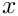and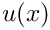at npts uniformly spaced points in the element to the specified file.
• The function Mesh::output_fct(...) plots the function specified by the function pointer in its last argument at the specified number of points in each of the constituent elements. This allows point-by-point comparisons between exact and FE solutions. Here we plot the exact solution at a larger number of points to ensure that the exact solution looks smooth even if only a small number of elements are used for the discretisation of the ODE.

Finally, we call the function Mesh::compute_error(...) which determines the square of the L2 error, based on the difference between the exact solution (specified by a function pointer) and the FE solution. We also plot the pointwise error in the specified output file.

//===start_of_doc=========================================================
/// Doc the solution in tecplot format. Label files with label.
//========================================================================
template<class ELEMENT>
void OneDPoissonProblem<ELEMENT>::doc_solution(const unsigned& label)
{
using namespace StringConversion;
// Number of plot points
unsigned npts;
npts=5;
// Output solution with specified number of plot points per element
ofstream solution_file(("soln" + to_string(label) + ".dat").c_str());
mesh_pt()->output(solution_file,npts);
solution_file.close();
// Output exact solution at much higher resolution (so we can
// see how well the solutions agree between nodal points)
ofstream exact_file(("exact_soln" + to_string(label) + ".dat").c_str());
mesh_pt()->output_fct(exact_file,20*npts,FishSolnOneDPoisson::get_exact_u);
exact_file.close();
// Doc pointwise error and compute norm of error and of the solution
double error,norm;
ofstream error_file(("error" + to_string(label) + ".dat").c_str());
mesh_pt()->compute_error(error_file,FishSolnOneDPoisson::get_exact_u,
error,norm);
error_file.close();
// Doc error norm:
cout << "\nNorm of error : " << sqrt(error) << std::endl;
cout << "Norm of solution : " << sqrt(norm) << std::endl << std::endl;
cout << std::endl;
} // end of doc

# Exercises

1. Run the code with different numbers of elements. How does the error between the exact and the analytical solution change?
2. Compare the error obtained with different element types – replace the four-node Poisson element, QPoissonElement<1,4>, by its lower-order counterparts QPoissonElement<1,3> and QPoissonElement<1,2>.
3. The fish-shaped exact solution (4) is fairly smooth. Postulate a more rapidly varying "exact" solution, such as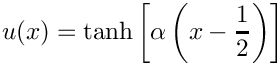which produces a "step" at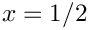when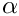becomes sufficiently large. Calculate the source function required for this function to be an exact solution and implement both functions in another namespace, TanhSolnOneDPoisson, say. Replace the reference to FishSolnOneDPoisson by TanhSolnOneDPoisson and repeat the above exercises.
4. Remove the Dirichlet boundary condition at the left end of the domain. What do you observe? [We shall return to this question in another example where we discuss the application of Neumann-type boundary conditions.]